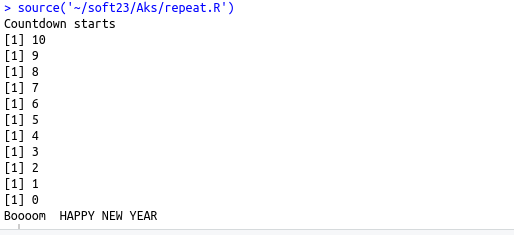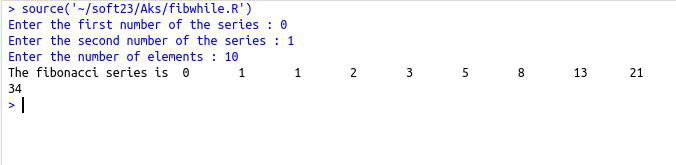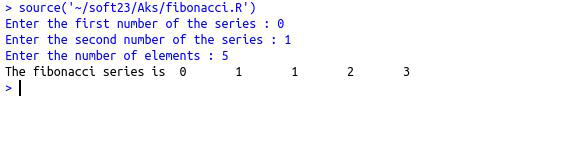• #5, First Floor, 4th Street Dr. Subbarayan Nagar Kodambakkam, Chennai-600 024 Landmark : Samiyar Madam
• pro@slogix.in
• +91- 81240 01111

#### Social List

##### Explain various Loops in R?
###### Description

To understand different looping concepts.

###### Process

Available loops:

• For
• While
• Repeat

For loop :

• Block of codes are executed until the given condition becomes FALSE.Condition is tested at the end of the loop body

Syntax :

for(i in vector)

{

Boy of the loop

}

While loop :

• Block of codes are executed until the given condition becomes FALSE.Condition is tested before executing a loop body.

Syntax :

While(Test expression)

{

Body of the loop

}

Repeat loop :

• Repeats a block of codes unconditionally.when the test expression becomes true the process is terminated.for termination process the keyword break is used

Syntax :

Repeat{

Commands

If(condition)

{

Break

}

• Loop control statements :
• Break – Terminates the loop statement and transfers execution to the statement immediately following the loop
• Next – Simulates the behaviour of R switch
###### Sapmle Code

Source code :(for loop)

x=as.integer(readline(prompt=”Enter the first number of the series : “))
y=as.integer(readline(prompt=”Enter the second number of the series : “))
n=as.integer(readline(prompt=”Enter the number of elements : “))
cat(“The fibonacci series is “,x,”\t”,y)
for(i in (1:(n-2)))
{
sum=x+y
x=y
y=sum
cat(“\t”,sum)
}

Source code :(While loop)

x=as.integer(readline(prompt=”Enter the first number of the series : “))
y=as.integer(readline(prompt=”Enter the second number of the series : “))
n=as.integer(readline(prompt=”Enter the number of elements : “))
cat(“The fibonacci series is “,x,”\t”,y)
i=0
while(i<(n-2))
{
sum=x+y
x=y
y=sum
cat(“\t”,sum)
i=i+1
}

Source code :(Repeat loop)

#repeat loop
i=10
cat(“Countdown starts\n”)
repeat{
print(i)
i=i-1
if(i==0)
{
break
}
}
cat(“Boooom\tHAPPY NEW YEAR”)

Source code :(Loop control)

a=c(1:10)
for ( i in a)
{
if (i ==5)
{
next
}
print(i)
}

###### Screenshots